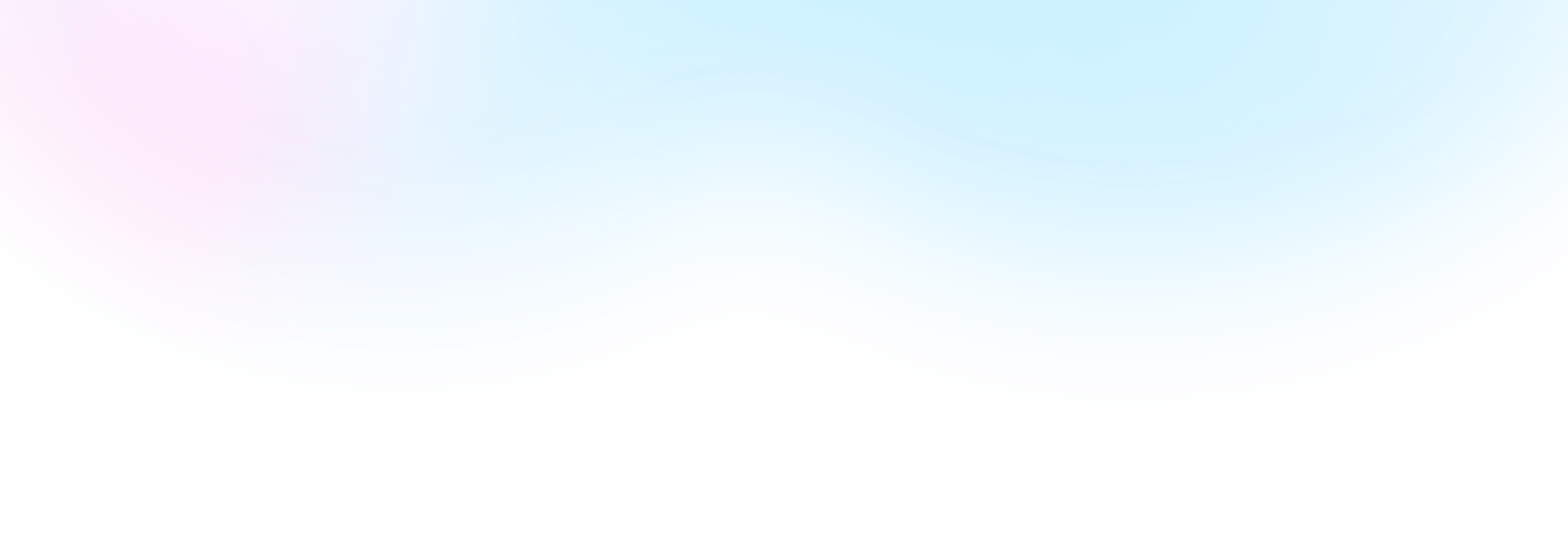Template Community / Worksheet For Kindergarten 1

# Worksheet For Kindergarten 1Kiraaaa
Published on 2022-02-10
Edit OnlineThe following worksheet is for kindergarten students to find the sum of two numbers and then color the picture accordingly. For instance, the first two numbers are two and seven. The kindergarten student will look at this worksheet and then try to find the summation of these two numbers. Once they have calculated the right number, they can write it down in the mentioned area. This way, they will learn the summation cool and engagingly. Similar to this, you can also create other worksheets for your kindergarten where they are tasked to do other mathematical equations, like subtraction, division, or multiplication.
Tag
science diagram
Mathematics Diagram
Report
0
20PostRecommended Templates